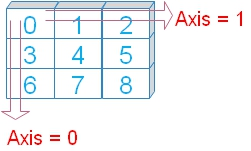# Pandas DataFrame min()

We can minimum number in rows or columns by using min().

``````import pandas as pd
my_dict={'NAME':['Ravi','Raju','Alex','Ron','King','Jack'],
'ID':[1,2,3,4,5,6],
'MATH':[80,40,70,70,70,30],
'ENGLISH':[80,70,40,50,60,30]}
my_data = pd.DataFrame(data=my_dict)
print(my_data.min())``````
Output
``````NAME       Alex
ID            1
MATH         30
ENGLISH      30``````
What is the minimum mark in MATH ?
``print(my_data['MATH'].max()) # 30 ``
We can get the row or details of the record who got minimum mark in MATH
``print(my_data[my_data['MATH'].min()==my_data['MATH']])``
Output is here
``````   NAME  ID  MATH  ENGLISH
5  Jack   6    30       30``````

## Using axisWe will use option axis=0 ( default ) by adding to above code.

( The last line is only changed )
``print(my_data.min(axis=1))``
Output is here
``````0    1
1    2
2    3
3    4
4    5
5    6``````
We are getting all the minimum values from the ID column. You can change the DataFrame and then check the minimum value.

## level option

For MultiIndex (hierarchical) axis we can specify the level.
``````import pandas as pd
my_dict=pd.MultiIndex.from_arrays(
[[1,2,3,4,5,6],
[80,40,70,70,70,30],
[80,70,40,50,60,30]],
names=['id','math','eng'])
my_data = pd.Series([4, 2, 0, 8,3,4], name='marks', index=my_dict)
print(my_data.min(level='math'))``````
Output
``````math
80    4
40    2
70    0
30    4``````

## Handling NA data using skipna option

We will use skipna=True to ignore the null or NA data. Let us check what happens if it is set to True ( skipna=True )
``````import numpy as np
import pandas as pd
my_dict={'NAME':['Ravi','Raju','Alex','Ron','King','Jack'],
'ID':[1,2,3,4,5,6],
'MATH':[80,40,70,70,70,30],
'ENGLISH':[80,70,np.nan,50,60,30]}
my_data = pd.DataFrame(data=my_dict)
print(my_data.min(skipna=True))``````
Output
``````NAME       Alex
ID            1
MATH         30
ENGLISH      30``````

## numeric_only

Default value is None, we can set it to True ( numeric_only=True ) to include only float, int, boolean columns. We can included all by setting it to False ( numeric_only=False ) . Let us see the outputs .
``print(my_data.min(numeric_only=False))``
Output is here
``````NAME       Alex
ID            1
MATH         30
ENGLISH      30``````

Subscribe to our YouTube Channel here

## Subscribe

* indicates required
Subscribe to plus2netplus2net.com